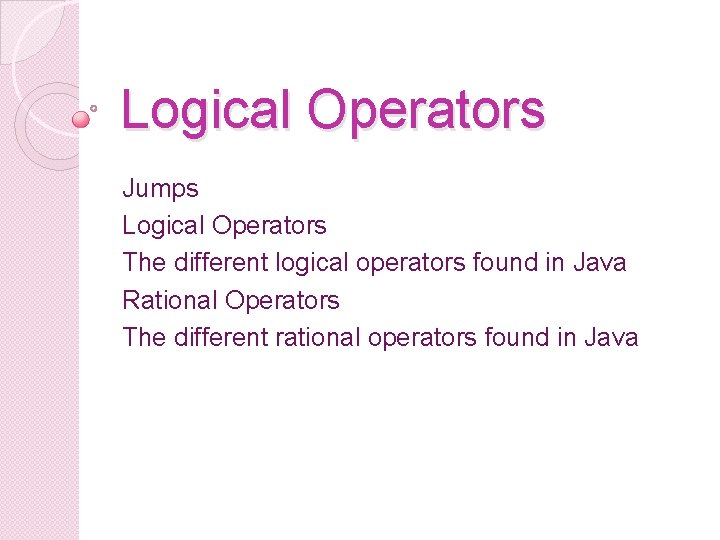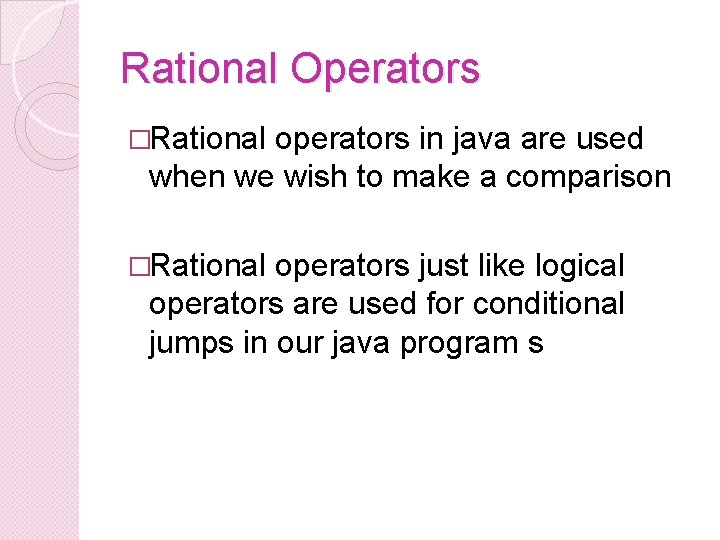# Logical Operators Jumps Logical Operators The different logical

• Slides: 8Logical Operators Jumps Logical Operators The different logical operators found in Java Rational Operators The different rational operators found in JavaJumps �A jump is also known as a branch �A jump is when you program skips certain steps to move on to another block of code �There are two types of jumps 1. Unconditional Jump = no condition is set 2. Conditional jump = a condition has toLogical Operators �Logical operators are functions used in Java �They are used to set certain conditions �These conditions are used when we have conditional jumps in our programsDifferent Logical Operators �There are many different operators, some of these include the following Logical Operator Meaning & Logical AND – both must be true | Logical OR – One or both must be true ^ Logical XOR – the NOT of the OR operator || Short Circuit OR – this stops when it finds the first true && Short Circuit AND – this stops when it finds the first true ! Logical Unary NOT – true becomes false and vice versaRational Operators �Rational operators in java are used when we wish to make a comparison �Rational operators just like logical operators are used for conditional jumps in our java program sDifferent Logical Operators Relational Meaning Operators == Equal To > Greater Than < Less Than >= Greater Than or Equal To <= Less Than or Equal ToIs the answer to these conditions true of false? 1. 2. 3. 4. 5. 6. 9 + 4 == 13 5*5 > 10 10 – 4 < 5 15 >= (10+5) 21 / 7 <= 4 12*2 != 24Is the answer to these conditions true of false? 1. 2. 3. 4. 5. 6. 9 + 4 == 13 False 5*5 > 10 True 10 – 4 < 5 True 15 >= (10+9) False 21 / 7 <= 4 True 12*2 != 24 False